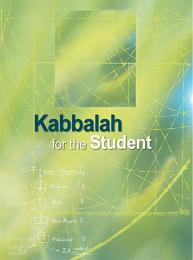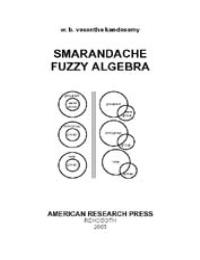My Account |   | HelpSearch Results (2 titles)

Searched over 7.2 Billion pages in 0.33 seconds

Cy Young (X) English (X) Non-Fiction (X)•### Kabbalah for the Student

##### By: Rav Michael Laitman

...ge of The Zohar for us and paved our way in it. Had he not passed away so young, it is hard to imagine the amount of Light that would be drawn out o... ...t, the world will come to its full correction. ThE f oUr A TTribUTES, mEr Cy, Tr UTh, jUSTiCE, And pEACE, in ThE individUAl And ThE CollECTivE Once ... ...b a l i s T s w r i T e a b o u T T h e w i s d o m o f Ka b b a l a h The young, or those who find themselves heavy and of little desire for the Inne... ... a great Mitzva (commandment, good deed) for the masses to study, old and young… 173 K a b b a l i s T s w r i T e a b o u T T h e w i s d o m o f ... ...hat and Remez is PR (pronounced Par), which is the root of Par Ben Bakar (young bull) in this world. And Pria and Revia (multiplication) come from t... ...d the book of Joshua.” What is it like? It is like a wealthy man who had a young son. One day, the man had to travel far away for many years. The ric...

•### Smarandache Fuzzy Algebra

##### By: W. B. Vasantha Kandasamy

..., λ) , x λ + y ν = (x + y) min (λ, v) , d µ o x λ + c K o y v = (dx + cy) min{µ , λ, k, v}. Proof: Left for the reader to refer . The... ...y for all x ∈ V, (K o A)(x) = sup{min {K(c), A(y)}c ∈ P ⊂ R , y ∈ V, x = cy P is a subfield in R relative to which V is defined}. DEFINITION 4.... .... x λ + y ν = (x + y) min (λ, ν) , d µ o x λ + c κ o y ν = (dx + cy) min{µ , λ, κ, ν}. Problem 4.5.59: Show if M is a δ-Noetherian module... ...r all x ∈ H ⊂ G (K o A)(x) = sup{min {K(c), A(y)}  c ∈ S, y ∈ H ⊂ G, x = cy}. Fuzzy singletons are defined as in case of any other fuzzy subset. ... ...,λ} , x λ + y ν = (x + y) min (λ ,ν) , dµ o x λ + c R o y ν = (dx + cy) min (µ, λ, k, ν} . Problem 5.5.39: Prove the fuzzy subset ν of SF (... ...49. JACOBSON, Nathan, Basic Algebra I, Freeman, New York, 1974. 50. JUN, Young Bae, NEGGERS, J. and KIM, Hee Sik, Normal L-fuzzy ideals in semiring... ...c groups, J. Fuzzy Math., 3, 465-470 (1995). 56. KIM, Kyung Ho, and JUN, Young Bae, Normal fuzzy R-subgroups in near- rings, Fuzzy Sets and Systems,... ...ximations in a fuzzy group, Inform. Sci., 90, 203-220 (1991). 71. KWON, Young-In, Fuzzy Near-ring modules over fuzzy near-rings, Pusan Kyongnam Ma...